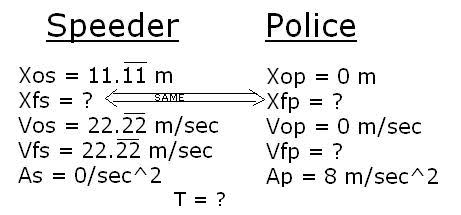# A Policeman Chases a Speeder

• Rker

## Homework Statement

A car is traveling at 80 km/hr in a school zone. A police car is at rest in the parking lot of the school. It takes the policeman 0.5 sec to react before he begins accelerating after the speeder with an acceleration of 8 m/sec2.

(a) How far has each car traveled when the police car catches the speeder?
(b) How fast is the police car going when it catches the speeder?
(c) Make a sketch of the position of both cars as a function of time?

## Homework EquationsThere may be different equation(s). These are just the main ones we're using for this unit.

## The Attempt at a SolutionMy setup is correct, but I'm having difficulty finding T. I tried plugging variables in equations, and it seems nothing's working. Once T is found, I'll hopefully be able to solve parts (a), (b), and (c) with ease.

As you have correctly indicated, Xf,s = Xf,p.

What does the formula for Xf give you in both cases? (Be especially careful about the value of Xi,p!)

When you set these formulas equal to each other, you will get a single equation for T.

Thank you for the fairly quick reply. I set the formulas equal to each other and got this:

0 = -4(m/sec^2)t^2 + 22.22(m/sec)t + 11.11m

Is that correct so far? And, if so, would I just use the quadratic formula to find positive T?

After a quick check, that looks like the correct answer. Just to be sure, can you tell me what you took for Xi,s and Xi,p (actually, you can take anything for Xi,s, for example -- all that matters is Xi,p - Xi,s)?

And you are completely right about solving it, too :)